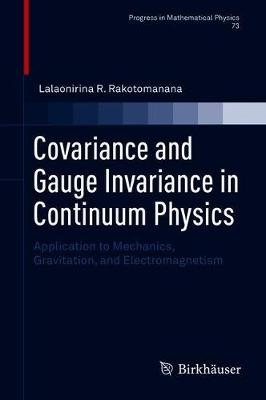•# Covariance and Gauge Invariance in Continuum Physics: Application to Mechanics, Gravitation, and Electromagnetism - Progress in Mathematical Physics 73 (Hardback)

(author)
£89.99
Hardback 325 Pages / Published: 12/07/2018
• We can order this

Usually dispatched within 3 weeks

This book presents a Lagrangian approach model to formulate various fields of continuum physics, ranging from gradient continuum elasticity to relativistic gravito-electromagnetism. It extends the classical theories based on Riemann geometry to Riemann-Cartan geometry, and then describes non-homogeneous continuum and spacetime with torsion in Einstein-Cartan relativistic gravitation.

It investigates two aspects of invariance of the Lagrangian: covariance of formulation following the method of Lovelock and Rund, and gauge invariance where the active diffeomorphism invariance is considered by using local Poincare gauge theory according to the Utiyama method.

Further, it develops various extensions of strain gradient continuum elasticity, relativistic gravitation and electromagnetism when the torsion field of the Riemann-Cartan continuum is not equal to zero. Lastly, it derives heterogeneous wave propagation equations within twisted and curved manifolds and proposes a relation between electromagnetic potential and torsion tensor.

Publisher: Birkhauser Verlag AG
ISBN: 9783319917818
Number of pages: 325
Weight: 676 g
Dimensions: 235 x 155 mm
Edition: 1st ed. 2018